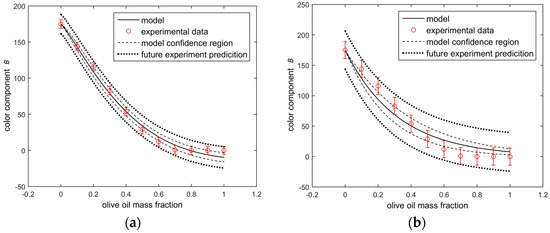Next Article in Journal
Does a Fractal Microstructure Require a Fractional Viscoelastic Model?
Next Article in Special Issue
The Craft of Fractional Modeling in Science and Engineering 2017
Previous Article in Journal / Special Issue
Fractional Derivatives with the Power-Law and the Mittag–Leffler Kernel Applied to the Nonlinear Baggs–Freedman Model

## Printed Edition

A printed edition of this Special Issue is available at MDPI Books....
Open AccessArticle

# Monitoring Liquid-Liquid Mixtures Using Fractional Calculus and Image Analysis

byErvin K. Lenzi 1,Andrea Ryba 2 andMarcelo K. Lenzi 3,*1
Departamento de Física, Universidade Estadual de Ponta Grossa, Av. Carlos Cavalcanti, 4748, Ponta Grossa 84030-900, Paraná, Brazil
2
Departamento de Transportes, Universidade Federal do Paraná, Rua Coronel Francisco H. dos Santos, 100, Curitiba 81531-980, Parana, Brazil
3
Departamento de Engenharia Química, Universidade Federal do Paraná, Rua Coronel Francisco H. dos Santos, 100, Curitiba 81531-980, Parana, Brazil
*
Author to whom correspondence should be addressed.
Fractal Fract 2018, 2(1), 11; https://doi.org/10.3390/fractalfract2010011
Received: 28 December 2017 / Revised: 6 February 2018 / Accepted: 8 February 2018 / Published: 11 February 2018
(This article belongs to the Special Issue The Craft of Fractional Modelling in Science and Engineering)
A fractional-calculus-based model is used to analyze the data obtained from the image analysis of mixtures of olive and soybean oil, which were quantified with the RGB color system. The model consists in a linear fractional differential equation, containing one fractional derivative of order α and an additional term multiplied by a parameter k. Using a hybrid parameter estimation scheme (genetic algorithm and a simplex-based algorithm), the model parameters were estimated as k = 3.42 ± 0.12 and α = 1.196 ± 0.027, while a correlation coefficient value of 0.997 was obtained. For the sake of comparison, parameter α was set equal to 1 and an integer order model was also studied, resulting in a one-parameter model with k = 3.11 ± 0.28. Joint confidence regions are calculated for the fractional order model, showing that the derivative order is statistically different from 1. Finally, an independent validation sample of color component B equal to 96 obtained from a sample with olive oil mass fraction equal to 0.25 is used for prediction purposes. The fractional model predicted the color B value equal to 93.1 ± 6.6. View Full-Text
Show FiguresFigure 1

MDPI and ACS Style

Lenzi, E.K.; Ryba, A.; Lenzi, M.K. Monitoring Liquid-Liquid Mixtures Using Fractional Calculus and Image Analysis. Fractal Fract 2018, 2, 11.